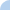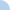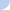# 1/24 Quia Homework

Difficulty level 1: Numbers 1-4 are very simple; just put the slope and y-intercept into the slope-intercept form.

Difficulty level 2: Numbers 5-7 give a point and a slope. Do the work required to find the equation of the line in slope-intercept form.

Difficulty level 3: Numbers 8-10 give two points. Find the slope using these two points and then do the work required to find the equation of the line in slope-intercept form.Quiz Log InThis activity was created by a Quia Web subscriber. Learn more about QuiaCreate your own activities